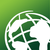# 3D intersection of 2 3D polylines with Python

1842
5
07-03-2018 06:48 AMNew Contributor

I am new to the arcpy. I searched the community and I didn't find the answer to the question.

I have problem with finding the intersection of two 3D polylines (each one with 2 vertex) with python in arcgis standard 10.5.  Polylines are not in a .shp file, because they created using polyline1 = arcpy.polyline(...) so I can call them directly.

Using point = polyline1.intersect(polyline2 , 1)  just create a multipoint class without anypoint in it.

I need the intersection point coordination (with z) as an answer

Also, I have problem with writing 3d polylines to .shp and .mdb files. after writing polylines to the file, the arcmap omit the z values and all the lines are horizontal.

So I really appriciate help with these 2 questions.

This is an example of the problems:

from os.path import expanduser
from os.path import join as joinadd
import os
import arcpy

#first question:

#2 below polylines obviously have intersection

#creating 3d polylines

polytemp1=arcpy.Polyline(arcpy.Array([arcpy.Point(1,2,1),arcpy.Point(10,11,10)]),"Unknown",True,False)

polytemp2=arcpy.Polyline(arcpy.Array([arcpy.Point(1,2,5),arcpy.Point(10,11,5)]),"Unknown",True,False)

#check for the intersection with disjoint

polytemp1.disjoint(polytemp2) #The answer is False, which means there is an intersection, but is disjoint check in 3D???? or just 2D????

#finding the point (3D)

pnt = polyline1.intersect(polyline2 , 1)

print 'newpoint' ,newpoint.pointCount, newpoint.type, newpoint.partCount #This shows that there is no point in the multipoint!!

#second question: writing a 3d polyline in a .shp file:

cursor.insertRow([polyline1])

del cursor

#now I have the .shp file, but the lines are 2D!!

Tags (4)
5 RepliesbyMVP Honored Contributor

Question 1: change one of the z coordinates so that the lines clearly do not intersect along the z-axis, but still would intersect along the xy plane, to check.

Question 2: you go through a lot of work to create a feature class called 'homeadd', and then insert into a different feature class, 'polyline1add'. Is that intentional?New Contributor

Thanks for the response Darren.

As I understand, .disjoint and .intersect just works in 2D (without Z).

Now the question is, 1. how to check if intersection exist in 3D?  2.How to find the intersection point (X,Y,Z)?byMVP Honored Contributor

I suppose you could test if the lines intersect in 2D. If so, find the XY coordinates where they intersect. Calculate the Z coordinate at those locations. See if they're the same. If the lines don't intersect in 2D, they don't intersect in 3D.New Contributor

The problem is If the lines have intersect in their 2D projection, it does not mean that they have intersect in 3D.byMVP Honored Contributor

That's why I suggest calculate the Z-coordinates at the 2D intersection point. If it's the same for both line, then they intersect 3D.